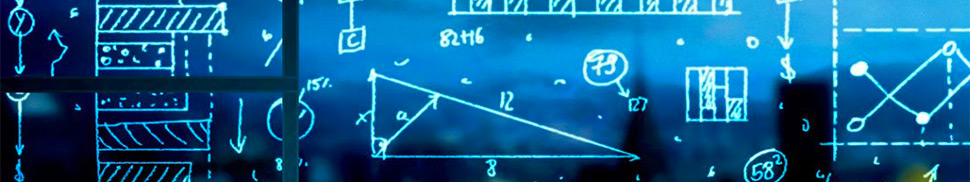# Institute of Mathematics### Teaching Profile

The institute offers the Faculty of Mechanical Engeneering students the following basic courses in mathematics: linear algebra, analytic geometry, calculus, numerical methods, constructive geometry and computer graphics, differential equations, infinite series, probability and mathematical statistics. Further mathematical courses, especially those of applied nature, are included in Master's and Doctor's degree programmes of individual specialisations.
Next the institute offers a Mathematical Engineering study designed to equip the graduates with a more profound theoretical engineering background in mathematics, programming, and state-of-the-art mathematical software.

### Scientific Profile

Research conducted at the institute focuses on applied mathematical analysis (finite element method, numerical solutions of differential equations), applied algebra and discrete mathematics, differential geometry and its applications, optimisation of engineering processes, mathematical modelling of forming processes, and digital image processing.
A number of staff members are in research teams at other faculty and university departments. The Institute co-operates with other Czech universities (Masaryk University Brno, Charles University in Prague) as well as with other non-university institutions such as the Dukovany Nuclear Power Plant. A wealth of cooperation is going on with universities abroad (Texas University of Austin, L'Aquila University (Italy), Technische Universität Hamburg, Universytet Jagiellonski (Krakow), University of Malta, Technische Universität Wien, Universität Potsdam, etc.)
The Institute staff members are involved in numerous research activities as testified by a large number of papers frequently published in renowned mathematical journals abroad and regular participation in international scientific conferences. Most of the staff work on research projects that receive funding from grants (mostly from the Grant Agency of the Czech Republic or the Ministry of Education as research designs) some of the staff members are even team leaders.
The Mathematical Engineering Doctor's degree programme at the Institute is based on the specialisations of the research conducted thus offering the doctoral students courses in applied mathematical analysis, statistics, and fuzzy mathematics, optimisation methods, and computer graphics.

DISPLAY MORETechnická 2

616 69 Brno